Chapter 9

As we know, the electromagnetic field energy density is: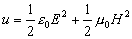(9.1)

Thus the electron electromagnetic field energy is: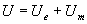(9.2)

Combine equation (7.5) and (8.4), thus: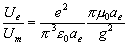(9.3)

Thus: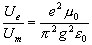(9.4)

As we know: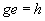(9.5)

And also: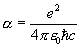(9.6)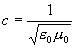(9.7)

Thus: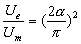(9.8)

Thus: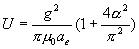(9.9)

Thus: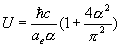(9.10)

As we know, the electron mass is one of the basic properties of electrons. Where does the electron mass come from? One of the most popular theories is the electromagnetic origin of electron mass.

From the above equation (9.10), we get the electron’s electromagnetic energy; let us make an assumption here that the electron’s mass origin is from the electromagnetic field energy, thus: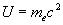(9.11)

In which U is the electron electromagnetic field energy, and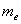is the electron mass, then combine the equation (9.10) and (9.11), we can get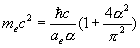(9.12)

Thus: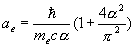(9.13)

And we know that the Bohr radius is: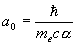(9.14)

Thus: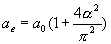(9.15)

As we know, the value of fine structure constant is about: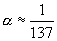(9.16)

Thus: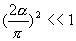(9.17)

Thus: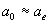(9.18)

Reference

Eur. J. Phys. 24 (May 2003) 271-275

General relativistic models for the electron

S. M. Blinder

Rept.Math.Phys. 47 (2001) 279-285

About Zitterbewegung and Electron Structure

Waldyr A. Rodrigues, Jayme Vaz, Erasmo Recami, and Giovanni Salesi

Physics Letters B 318 (1993) 623-628

Zitterbewegung and the Electromagnetic Field of the Electron

J.Vaz Jr e W.A.Rodrigues Jr,

Physics Letters B, 319, 203-208, (1993).

Spin and Electron Structure

M.Pavsic, E.Recami, W.A.Rodrigues Jr, D.G.Maccarrone, F.Raciti e G.Salesi,

Physics Letters B, 318, 481-488, (1993).

Mass and Spin Renormalization in Lorentz Electrodynamics

Walter Appel, Michael K.-H. Kiessling

Annals Phys. 289 (2001) 24-83

An Elementary Account of the Factor of 4/3 in the Electromagnetic Mass

P. Moylan

Am. J. Phys. 63, 818-820 (1995).

The Electromagnetic Mass of a Charged Particle

V.A. Kuligin, G.A. Kuligina, M.V. Korneva

APEIRON Vol. 3 Nr. 1 January 1996